Aplikace

# ARF (average reduced frequency)

ARF1) is one of the many adjusted frequencies of a word form in a corpus. Adjusted frequencies adjust the simple frequency (number of occurrences) of a given word or phenomenon in the corpus to the degree of the uniformity of how its occurrences are distributed, taking into account dispersion. ARF helps prevent a scenario where frequency lists attach significance to words which are contained only in one text but with many individual occurrences, whereas in the rest of the corpus (and the language) they are much less common.

## Reduced frequency and ARF

Let us assume that we have found in the corpus two words with the same occurrence rate. The first of these two words is found only in one single document, whereas the other is more or less evenly distributed throughout the entire corpus. In all probability, the second word will be more common than the first, but the occurrence rate will not tell us that. This is why we introduce the so-called reduced frequency.

Its definition is as follows: We use the letter f to label the frequency of a given word in the corpus. We divide the positions in the entire corpus into f sections of equal size. If the total number of words in the corpus should be divisible by f, the sections would be the same size; in the opposite case they may differ in one position. A reduced frequency is then the number of sections in which the given word occurs at least once.

The first word from our example will have a reduced frequency of either 1 (if all of its occurrences fall under on section) or 2 (if the boundary between two sections should happen to be in the middle of a cluster of occurrences. The second word will have a much higher value for reduced frequency. In extremely unlikely cases the reduced frequency could theoretically be the same as the frequency, which would happen should every occurrence of a given word fall under one single section. This very rarely happens in reality, especially as far as words with higher frequencies are concerned.

The average reduced frequency (ARF) is then derived from the reduced frequency in the sense that it takes into account all possible compilations of the corpus (the order of the texts in it). It is calculated as an average of the reduced frequency from all possible compilations of the corpus.

## ARF calculations

The value of ARF is given by

$$ARF = \frac{1}{v} \sum_{i=1}^{f} \min (d_{i}, v)$$

where $f$ is the frequency of the given expression in a corpus of the size $N$, $d_{i}$ are the distances between the individual occurrences of this expression in the corpus (the number of words lying between them) and $v$ is the average distance between its occurrences and is given by $v = \frac{N}{f}$.

## ARF values

Because N is divisible by f only very rarely, the ARF typically takes on fractional values, which is common for adjusted frequencies. The ARF value for a given expression is a correction of its frequency based on the distribution of its occurrence is the corpus: the more even the distribution, the closer the ARF value will be to the frequency and vice versa; for expressions whose occurrences center around one cluster in the corpus, the ARF will be close to 1 regardless of frequency.

The maximum ARF value is equal to the frequency (if $d_{i} = v$ for all $i$, i.e. if the distance between all occurrences of the expression is the same), and its lowest possible value is equal to 1.

The value of ARF for high frequency expressions with an even distribution of occurrences is approximately a third of their frequency (but specifically only for frequencies over 50 000), however for technical terms occurring only in several documents it can be significantly (10 to 100 times) lower than the frequency. ARF is in comparison to the frequency much less sensitive to the (non-)inclusion of specific texts in the corpus, and therefore corresponds better to the intuitive understanding of “common words”.

ARF became known in the Czech environment thanks to its implementation in the former corpus manager Manatee/Bonito (today in the KonText interface), and did well in comparison with other commonly used adjusted frequencies and dispersion rates.2) Apart from this, the ARF was proven to work in practice as the main criterion for determining word commonness in the compilation of both the newest frequency dictionaries of Czech.

M. Křen, V. Cvrček

1)
Savický, P. & J. Hlaváčová: Measures of Word Commonness. In Journal of Quantitative Linguistics 9, 2002, 215–231. (preliminary version)
2)
Gries, S. T.: Dispersions and adjusted frequencies in corpora. In International Journal of Corpus Linguistics 13, 2008, 403–437.
•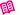Manuály
•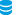Korpusy ČNK
•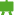Kurz práce s ČNK
•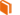Slovníček pojmů
•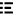Seznamy